# Radioactive Decay, Nuclear ReactionsPage 1

#### WATCH ALL SLIDES

Slide 1## Nuclear Reactions

Alpha, Beta, and Gamma Decay

Slide 2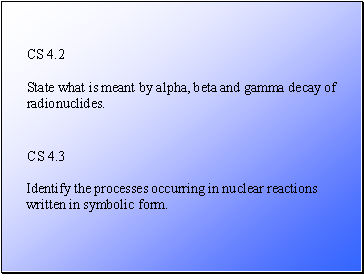CS 4.2

CS 4.3

State what is meant by alpha, beta and gamma decay of radionuclides.

Identify the processes occurring in nuclear reactions written in symbolic form.

Slide 3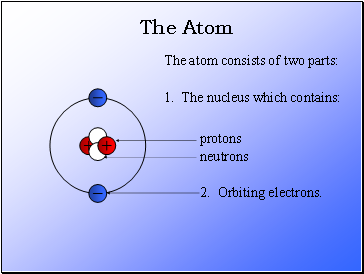## The Atom

The atom consists of two parts:

1. The nucleus which contains:

2. Orbiting electrons.

protons

neutrons

Slide 4All matter is made up of elements (e.g. carbon, hydrogen, etc.).

The smallest part of an element is called an atom.

Atom of different elements contain different numbers of protons.

The mass of an atom is almost entirely due to the number of protons and neutrons.

The Atom

Slide 5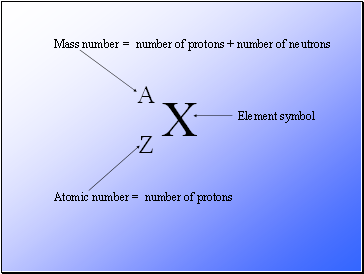X

A

Z

Mass number

Atomic number

Element symbol

= number of protons + number of neutrons

= number of protons

Slide 6A = number of protons + number of neutrons

Z = number of protons

A – Z = number of neutrons

Number of neutrons = Mass Number – Atomic Number

Slide 7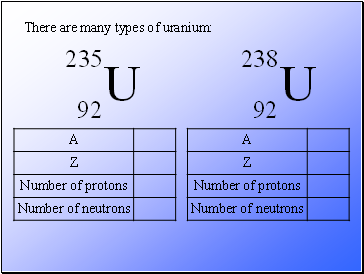There are many types of uranium:

Slide 8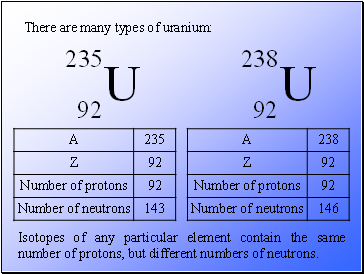There are many types of uranium:

Isotopes of any particular element contain the same number of protons, but different numbers of neutrons.

Slide 9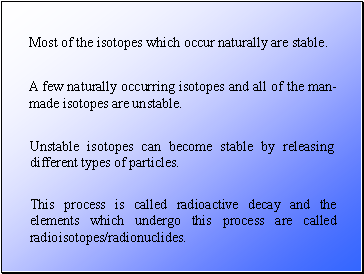Most of the isotopes which occur naturally are stable.

A few naturally occurring isotopes and all of the man-made isotopes are unstable.

Unstable isotopes can become stable by releasing different types of particles.

Slide 10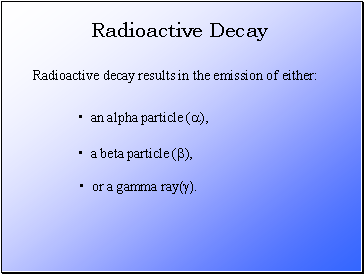Radioactive decay results in the emission of either:

an alpha particle (a),

a beta particle (b),

or a gamma ray(g).

Slide 11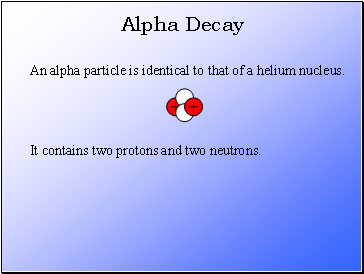## Alpha Decay

An alpha particle is identical to that of a helium nucleus.

It contains two protons and two neutrons.

Slide 12Go to page:
1  2